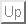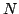Next: Polynomial systems

• La page de J-P. Merlet
• La page Présentation de COPRIN
• La page "Présentation" de l'INRIA

The COPRIN examples page

Most of the problems presented in this document have been submitted to the ALIAS-C++ library through the ALIAS-Maple library, a software developed in the COPRIN project. A problem with the prefix AOL has been submitted to the on-line solver available at the home page of the COPRIN project.

Some of these problems have been solved using Maple procedures based on the intpakX package:

```http://www.math.uni-wuppertal.de/~xsc/software/intpakX/d_l_c.html
```
i.e. the multi-precision interval arithmetic library developed at the Department of Mathematics and Computer Science, University of Wuppertal.

• polynomial system solving
• non polynomial system solving
• optimization problems
• miscellaneous problems: parametric polynomials, inequalities,...

In the last section 6 problems that are considered to be difficult: here difficult means that the computation time for a system with a fixed number of unknowns on a single computer is larger than 10mn or, if the number of equations is variable, that the computation time is larger than 10mn for a system with 10 equations.

Warning:

1. Naming: some systems are dependent upon an integerwhose value indicates the complexity of the system. In that case computation times for variousare given in the corresponding section. But this is not sufficient for our domain because a given system admits various mathematically equivalent forms (that may be obtained for example by combining the equations) and the solving time is heavily dependent upon the form that is provided to the solver. In this document the naming is done according to the following rules:
• the generic name of the system: the system that is solved is exactly the one described in the reference
• the generic name of the system with a version number: the system that is solved is a variant of the original system (which is usually simpler to solve)
Hence the equations that are presented in a section are exactly the one that are solved without any pre-processing (except maybe for a conversion into Horner form).
2. Exact solutions: in this document exact solution implies that the algorithm was able to determine that there was a unique solution within some ranges for the unknowns, solution that can be safely computed with an iterative scheme up to an arbitrary accuracy.
3. Solving procedure: name of methods indicated in typewriter font refers to procedure of the ALIAS maple library.
4. Computation times: they indicate the solving time of the C++ code generated by the ALIAS Maple interface without any processing of the generated C++ code and with standard settings for the solving parameters. We just allow a choice in the solving procedure among the various solvers provided by ALIAS-Maple. As for the filtering heuristics (such as 2B, 3B,...) we allow only the one implemented in ALIAS-Maple and most of the time we use the same heuristics. Hence they are indicative only as a specific treatment of the equations or fine tuning of the solving parameters will in many cases allows to decrease drastically the solving time. Hence the presented solving times do not fully reflect what maximal performance can be obtained with interval analysis for a specific system. Different computation times may be indicated corresponding to progress in the ALIAS solvers
5. Solutions set: computation times are indicated for finding all the solutions of the systems within the indicated search space. In most cases the solutions are certified (denoted exact in the examples) i.e.:
• for equations systems we have found an interval for the unknowns in which we can guarantee that there is one and only one solution, solution that we can compute with an arbitrary accuracy (the computation time includes the computation of the solution with an accuracy that is usually 1e-6)
• for optimization problems we have found an approximation of the global extrema such that the distance between approximation and the extrema is less than a given threshold (usually 1e-6)

This page is frequently updated either with new examples or with improvement in the ALIAS computation time.

Contact: Jean-Pierre.Merlet@sophia.inria.fr

Updated on: December 4, 2007

Number of examples: 131Next: Polynomial systems
• La page de J-P. Merlet